Sérgio O. Parreiras

## Using Reduce to Compute Nash EquilibriaNBCDFPDF

### Classroom Tools for Game Theory

The Karush-Kuhn-Tucker equations (under suitable conditions) provide necessary and sufficient conditions for the solution of the problem of maximizing (minimizing) a concave (convex) function. This article corrects the program in , which computes the solution of Karush-Kuhn-Tucker equations. Our main goal, however, is to provide a program to compute the set of all Nash equilibria of a bimatrix game. The program works well for “small” games (i.e. 4×4 or smaller games); thus, in particular, it is suitable for constructing classroom examples and as an additional tool to empower students in classes using game theory.

### Karush-Kuhn-Tucker Equations

The Karush-Kuhn-Tucker equations (under suitable assumptions) provide necessary and sufficient conditions for the solution of the problem of maximizing (minimizing) a concave (convex) function.

For an excellent reference, see the tutorial in . Here we modify the code of  by correcting minor typos, simplifying, and letting the user specify restrictions on the exogenous parameters of the model.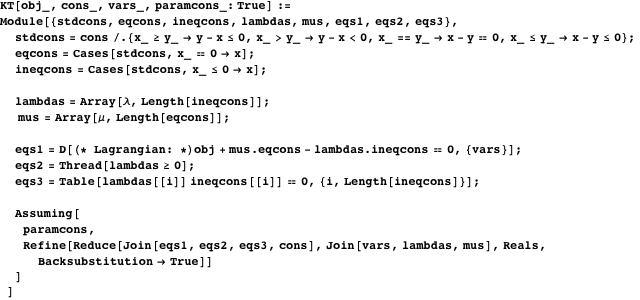#### An Example from Consumer Choice

The inputs of KT are the objective function to be maximized, the list of constraints, and the list of choice variables. Here is an example from consumer choice theory: maximize a utility function, subject to a budget constraint.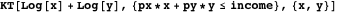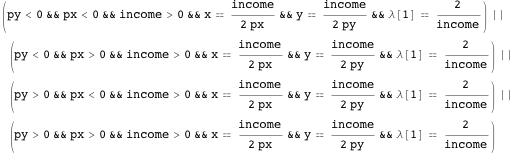Several of the solutions do not make economic sense, because they do not use the fact that the income, price of good, and price of goodare all positive. However, KT lets the user specify restrictions on the exogenous parameters of the model.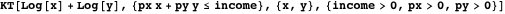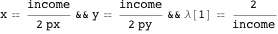An important advantage of KT over other optimization functions (such as Maximize or Minimize) is that KT returns the value of the Kuhn-Tucker multipliers. These multipliers have an important economic interpretation: they are shadow prices for the constrained resources. In the above example, for instance, the value of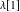is the “infinitesimal” increment in the utility function of the consumer that is generated when the budget constraint is relaxed by increasing the consumer’s income by an “infinitesimal amount.”

### Computing Nash Equilibrium of Bimatrix Games

Nash equilibrium is the main solution concept in game theory. It is a crucial tool for economics and political science models. Essentially, a Nash equilibrium is a profile of strategies (one strategy for each player), such that if a player takes the choices of the others as given (i.e. as parameters), then the player’s strategy must maximize his or her payoff.

The function Nash takes as input the payoff function of player 1, the payoff function of player 2, and the actions available to players 1 and 2. It returns the entire set of Nash equilibria.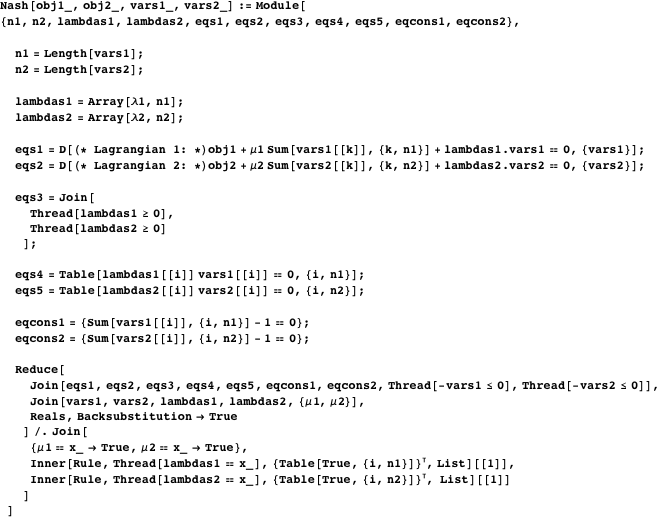#### A Colonel Blotto’s Game

There are many versions of Colonel Blotto’s game; this is a simple one taken from . General A (row player) has three divisions to defend a city; she has to choose how many divisions to place at the north road and how many divisions at the south road. General B (column player) has two divisions to try to invade the city; he also has to choose how many divisions to be assigned to the north road and how many to the south road. If General A has at least as many divisions as General B at a given road, General A wins the battle there (defense is favored in the case of a tie). To win the game, however, A must defeat B on both battlefields. Thus, A has four possible strategies and B has three strategies. The table below summarizes the players’ strategies and payoffs (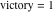,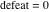for the whole campaign). For example, in the first row and first column the entry is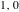, which means A won and B lost; A chose three divisions for the north road and none for the south road; B chose two for the north and none for the south. Because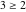and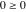, A won both battles.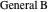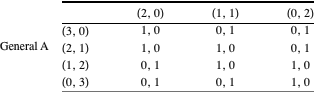Game 1. Colonel Blotto.

A Nash equilibrium for this game is a probability distribution over strategies; use P for the probabilities chosen by General A and Q for the probabilities chosen by General B.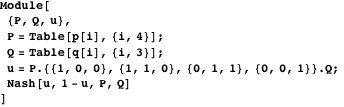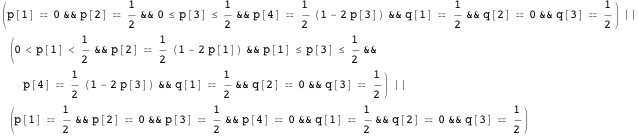The game has many Nash equilibria, but we still can make predictions: General B is never going to spread his forces evenly (the probability of his second strategy is zero in any equilibrium,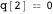); with probability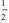, B’s two divisions are placed at the north road (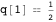) and with probability, they are placed at the south road (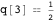). As for General A, the probability that she places all of her three divisions on one front is less than half (i.e.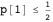and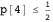). Also, the probability that General A places two or more divisions at the north (or south) is always equal to half (i.e.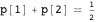and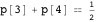).

#### A Card Game

This game is also borrowed from . A deck has two cards, one high and one low. Each player places one dollar into the pot. Player 1 gets one card from the deck. Player 2 does not see Player 1’s card. Player 1 decides whether to raise (by placing another dollar in the pot) or not raise. Player 2 observes 1’s action and then has to decide whether to match the bet or fold. If Player 2 folds, then Player 1 wins the contents of the pot. However, if Player 2 matches, Player 2 places another dollar into the pot if Player 1 had previously raised. Player 1 reveals her card. If it is the high card, Player 1 wins the pot; otherwise, Player 2 wins it.

See Figure 1 for the corresponding game tree. We introduce a fictitious player, Nature, who randomly decides if the card is high or low. We depict the bimatrix representation of the game. Player 1 has four strategies: always raise (RR), always not raise (NN), raise if the card is high and not otherwise (RN), and not raise if the card is high and raise otherwise (NR). Player 2 also has four strategies: always match (MM), always fold (FF), match only if Player 1 raised (MF), and fold only if Player 1 raised (FM). For simplicity, in the bimatrix representation, we write the expected payoffs of Player 1 and omit Player 2’s payoffs (this is without loss of generality in zero-sum games).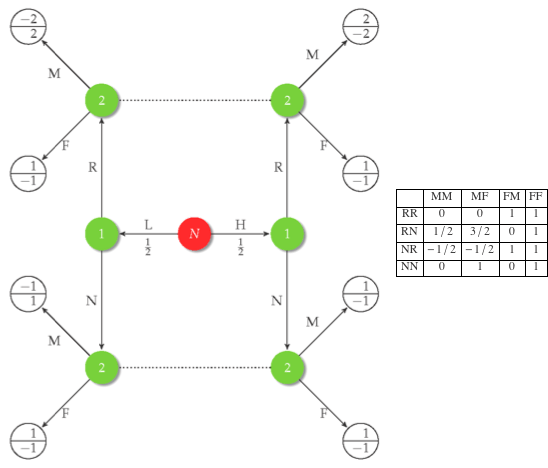Figure 1. Game tree of the card game.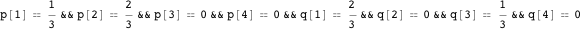In this case, the Nash equilibrium delivers a sharp prediction. When Player 1 has the high card, she always raises (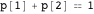), but when she has the low card, she bluffs with probability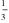(the probability of RR is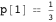). When Player 1 does not raise, Player 2 always matches (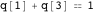). If Player 1 raises, Player 2 still may match, but with probability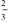(the probability of always matching MM is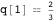).

### Summary

We extended the code of  to solve for Kuhn-Tucker conditions with additional assumptions on parameters and, more importantly, using the Kuhn-Tucker equations we provide a program to compute all the Nash equilibria of finite bimatrix games.

### Conclusion

We presented a program to compute the set of all Nash equilibria in finite bimatrix games. Its intended goal is as a classroom tool for students and instructors. Needless to say, the code is not efficient. For larger inputs (say bimatrix games with five or more actions per player), Reduce often fails to solve the system of Kuhn-Tucker equations. For optimizing algorithms, we suggest . Nevertheless, with continuous improvement of hardware and algorithms for solving semialgebraic systems (see ), these methods may become useful for research applications sooner than we think. Finally, as algorithmic game theory courses become more popular in computer science departments, it seems that the time to bring computational methods and algorithms to economics departments is already overdue.

### References

  F. J. Kampas, “Tricks of Using Reduce to Solve Khun-Tucker Equations,” The Mathematica Journal, 9(4), 2005 pp. 686-689. www.mathematica-journal.com/issue/v9i4/contents/Tricks9-4/Tricks9-4_ 2.html.  M. J. Osborne. “Optimization: The Kuhn-Tucker Conditions for Problems with Inequality Constraints,” from Mathematical Methods for Economic Theory: A Tutorial. (Jan 8, 2014) www.economics.utoronto.ca/osborne/MathTutorial/MOIF.HTM.  M. Osborne, “An Introduction to Game Theory,” New York: Oxford University Press, 2004.  R. D. McKelvey, A. M. McLennan, and T. L. Turocy. “Gambit: Software Tools for Game Theory.” (Jan 8, 2014) www.gambit-project.org.  Wolfram Research, “Real Polynomial Systems” from Wolfram Mathematica Documentation Center—A Wolfram Web Resource. reference.wolfram.com/mathematica/tutorial/RealPolynomialSystems.html. S. O. Parreiras, “Using Reduce to Compute Nash Equilibria,” The Mathematica Journal, 2014. dx.doi.org/doi:10.3888/tmj.16-3.